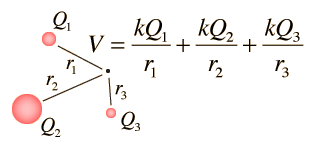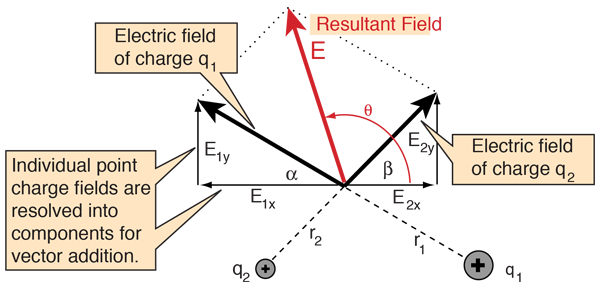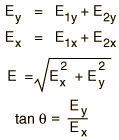# Multiple Point Charges

The electric potential (voltage) at any point in space produced by any number of point charges can be calculated from the point charge expression by simple addition since voltage is a scalar quantity. The potential from a continuous charge distribution can be obtained by summing the contributions from each point in the source charge.

The calculation of potential is inherently simpler than the vector sum required to calculate the electric field.The electric field outside a spherically symmetric charge distribution is identical to that of a point charge as can be shown by Gauss' Law. So the potential outside a spherical charge distribution is identical to that of a point charge.
 Calculation
 Potentials for other charge geometries
Index

Voltage concepts

 HyperPhysics***** Electricity and Magnetism R Nave
Go Back

# Multiple Point Charges

The electric field from multiple point charges can be obtained by taking the vector sum of the electric fields of the individual charges.Label diagram for calculation
 Calculation Voltage from point charges
Index

Electric field concepts

 HyperPhysics***** Electricity and Magnetism R Nave
Go Back

# Multiple Point Charges

The electric field from multiple point charges can be obtained by taking the vector sum of the electric fields of the individual charges.After calculating the individual point charge fields, their components must be found and added to form the components of the resultant field. The resultant electric field can then be put into polar form. Care must be taken to establish the correct quadrant for the angle because of ambiguities in the arctangent.Discussion of field diagram
 Calculation Voltage from point charges
Index

Electric field concepts

 HyperPhysics***** Electricity and Magnetism R Nave
Go Back